# B M VASU

•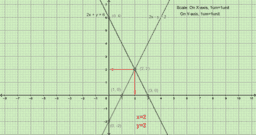### Solve 2x+y=6 & 2x-y=2 by Graph

Activity

B M VASU

•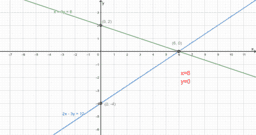### Solution of x+3y=6 & 2x-3y=12 by graph

Activity

B M VASU

•### graph

Activity

B M VASU

•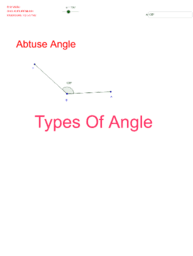### Types of Angle

Activity

B M VASU

•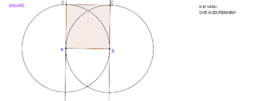### Square

Activity

B M VASU

•### Tracing of trigonometric ratios

Activity

B M VASU

•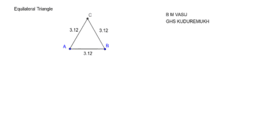Activity

B M VASU